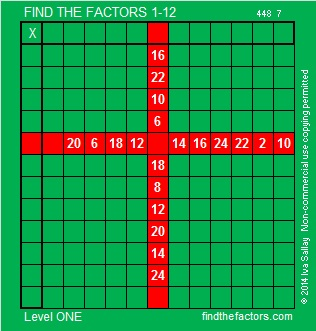# 310 and Level 1

• 310 is a composite number.
• Prime factorization: 310 = 2 x 5 x 31
• The exponents in the prime factorization are 1, 1, and 1. Adding one to each and multiplying we get (1 + 1)(1 + 1)(1 + 1) = 2 x 2 x 2 = 8. Therefore 310 has exactly 8 factors.
• Factors of 310: 1, 2, 5, 10, 31, 62, 155, 310
• Factor pairs: 310 = 1 x 310, 2 x 155, 5 x 62, or 10 x 31
• 310 has no square factors that allow its square root to be simplified. √310 ≈ 17.607This simply wrapped puzzle gift box is very easy to unravel.Print the puzzles or type the factors on this excel file: 12 Factors 2014-12-011.Paula
2.Joseph Nebus